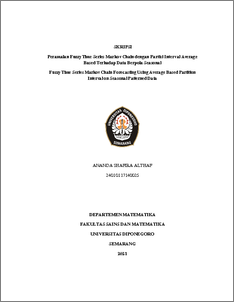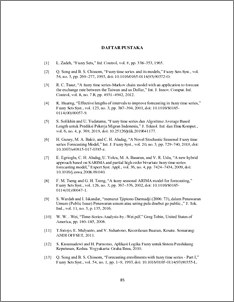# Fuzzy Time Series Markov Chain Forecasting Using Average Based Partition Interval on Seasonal Patterned Data

Althaf, Ananda Shafira (2021) Fuzzy Time Series Markov Chain Forecasting Using Average Based Partition Interval on Seasonal Patterned Data. Undergraduate thesis, UNDIP.Preview
PDF
475KbPDF
Restricted to Repository staff only

1261KbPreview
PDF
120Kb

## Abstract

Fuzzy time series Markov chain is a forecasting method by combining concepts fuzzy and Markov chains for time series data. Forecasting with this method uses interval partitioning on the universe of speech to form a set fuzzy. Interval partitioning can affect forecasting accuracy so that the universe set is partitioned using an average based which is an interval partitioning method based on the average approach. Fuzzy time series Markov chain with interval partition average based can be used for forecasting seasonal patterned data. By looking at and taking into account the period seasonal formed, it can affect the accuracy of forecasting. The solution of this method is obtained by fuzzifying historical data into sets fuzzy formed based on interval partitioning, determining relations fuzzy based on the period seasonal formed on ACF and PACF plots, forming relation groups fuzzy based on the same current state. Then calculate the forecast value based on the transition matrix, calculate the adjustment value so that the final forecasting result is obtained. From the calculation of the forecasting method fuzzy time series Markov chain with interval partitions average based on patterned data on case study Sydney’s average maximum temperature in 2011- 2020, it seasonal gives very good results based on the AFER and MSE value categories. Keywords: Forecasting, Fuzzy time series, Markov chain, Average based, Seasonal, ACF, PACF.

Item Type: Thesis (Undergraduate) Q Science > QA Mathematics Faculty of Science and Mathematics > Department of Mathematics 84174 INVALID USER 09 Jun 2022 21:57 09 Jun 2022 21:57

Repository Staff Only: item control page